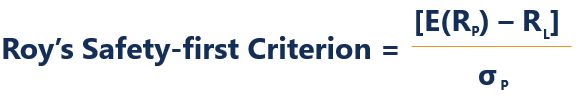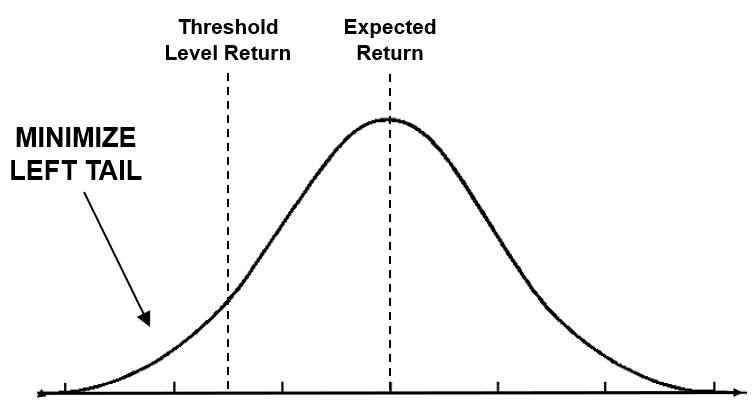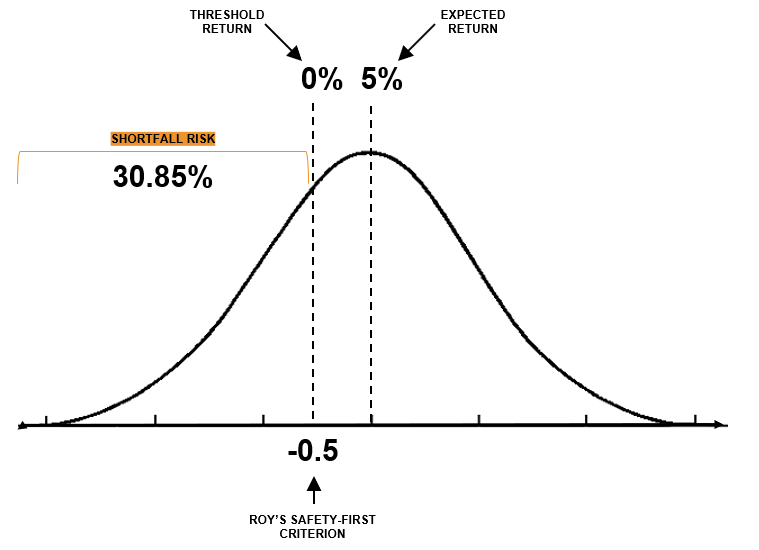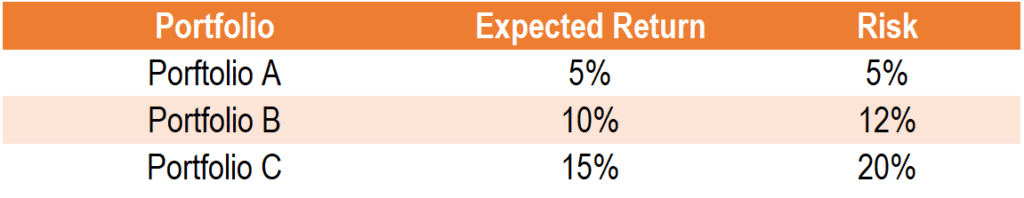# Roy’s Safety-first Criterion

A risk management technique based on the criterion that the probability of a portfolio’s return dropping below a threshold level return is reduced

## What is Roy’s Safety-first Criterion?

Roy’s safety-first criterion is a risk management technique used by investors to compare and choose a portfolio based on the criterion that the probability of a portfolio’s return dropping below a threshold level return is reduced.

In Roy’s safety-first criterion, the optimal portfolio is one that minimizes the probability of the portfolio’s return falling below a threshold return level. The portfolio with the highest Roy’s safety-first criterion has the lowest probability of a portfolio generating a return lower than the threshold level return.

### Summary:

• Roy’s safety-first criterion is used by investors to choose a portfolio based on the criterion that the probability of the portfolio’s return dropping below a threshold level is reduced.
• The value given by Roy’s safety-first criterion indicates the number of standard deviations below the mean.
• The formula for Roy’s safety-first criterion is [E(RP) – RL] / σp

### Formula for Roy’s Safety-first CriterionWhere:

• E(Rp) is the expected portfolio return;
• RL is the threshold level return (the minimum acceptable return); and
• σp is the standard deviation, or risk, of the portfolio.

Note that the formula for Roy’s safety-first criterion assumes that portfolio returns are normally distributed.

### Visual Representation of Roy’s Safety-first CriterionWhere:

• Expected Return is E(Rp); and
• Threshold Level Return is RL.

The goal of Roy’s safety-first criterion is to minimize the left tail. The area to the left of the threshold level return is the probability of the portfolio generating a return less than the threshold.

The value given by Roy’s safety-first criterion indicates the number of standard deviations below the mean. For example, a value of 1 indicates one standard deviation below the mean. Therefore, the higher the criterion value, the smaller the left tail and the lower the probability of the portfolio generating a return less than the threshold.

### Shortfall Risk and Roy’s Safety-first Criterion

Shortfall risk and Roy’s safety-first criterion goes hand-in-hand. Shortfall risk is the probability of generating a return lower than the threshold level return. In other words, shortfall risk is the area to the left of the threshold level return on a normal distribution graph. It is important to note that:

The higher the safety-first criterion, the lower the shortfall risk.

The lower the safety-first criterion, the higher the shortfall risk.

The shortfall risk can be calculated through a z-table for negative values. Below, we will do a comprehensive example.

For example, consider a portfolio with an expected return of 5%, a standard deviation of 10%, and a threshold return level of 0%. What are the Roy’s safety-first criterion and shortfall risk assuming that the portfolio is normally distributed?

The Roy’s safety-first criterion is calculated as (5% – 0%) / 10% = 0.5.Illustrated above, the expected return is 5%, the threshold return is 0%, and Roy’s safety-first criterion yields 0.5, which is 0.5 standard deviations below the expected return. Shortfall risk is the area under the curve starting from the left of the threshold return. Using a z-table for negative values, -0.5 corresponds to a z-score of 0.3085 or 30.85%.

### Example of Roy’s Safety-first Criterion

Consider three portfolios with the return and risk profiles provided below. Assume that the investor wants to minimize the probability of the portfolio returning less than 0%. In other words, the investor’s minimum acceptable return is 0%. Based on Roy’s safety-first criterion, which portfolio should the investor invest in?The Roy’s safety-first criterion for Portfolio A is calculated as (5% – 0%) / 5% = 1.

The Roy’s safety-first criterion for Portfolio B is calculated as (10% – 0%) / 12% = 0.83.

The Roy’s safety-first criterion for Portfolio C is calculated as (15% – 0%) / 20% = 0.75.

Based on Roy’s safety-first criterion, the ratio with the largest safety-first criterion has the lowest probability of getting a return less than 0%. In our example, it would be Portfolio A.

CFI is the official provider of the Financial Modeling and Valuation Analyst (FMVA)™ certification program, designed to transform anyone into a world-class financial analyst.

To keep learning and developing your knowledge of financial analysis, we highly recommend the additional resources below:

• Basis Risk
• Investing: A Beginner’s Guide
• Rate of Return
• Total Probability Rule

### Corporate Finance Training

Advance your career in investment banking, private equity, FP&A, treasury, corporate development and other areas of corporate finance.

Enroll in CFI’s Finance Courses to take your career to the next level! Learn step-by-step from professional Wall Street instructors today.